First Midterm Exam, M403K, Fall 2000

1. Consider the function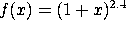a) Find f'(x).

b) Find the slope of the line tangent to the curve y=f(x) at x=0.

c) Find the equation of this tangent line.

d) Use this tangent line to approximate f(0.01).

2. From the following table, estimate f'(1). Indicate clearly how you obtain your answer: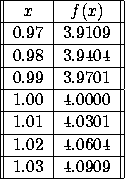3. Evaluate the following limits, if they exist (or write DNE if they do not).

a)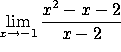b)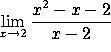c)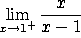d)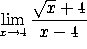4. Take the derivatives of the following functions with respect to x. You do not need to simplify your answers:

a)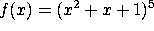b)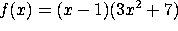c)d)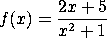5. New England Widget Technologies (NEWT) makes expensive high-tech widgets. Their marketing department has determined that the demand function is x = 4000 - 2p, where x is the number of widgets sold and p is the price. Their cost function is C(x)= 200,000 + 1000x.

a) Find the price p(x) and the revenue R(x) as a function of x.

b) Compute the marginal cost, marginal revenue and marginal profit as a function of x.

c) The company has a current production level of x=1500. To increase revenue, should the company increase or decrease production? [Note: you do not need to compute the optimal level of production. You just need to say whether it is higher or lower than 1500.]

c) The company has a current production level of x=1500. To increase profit, should the company increase or decrease production?